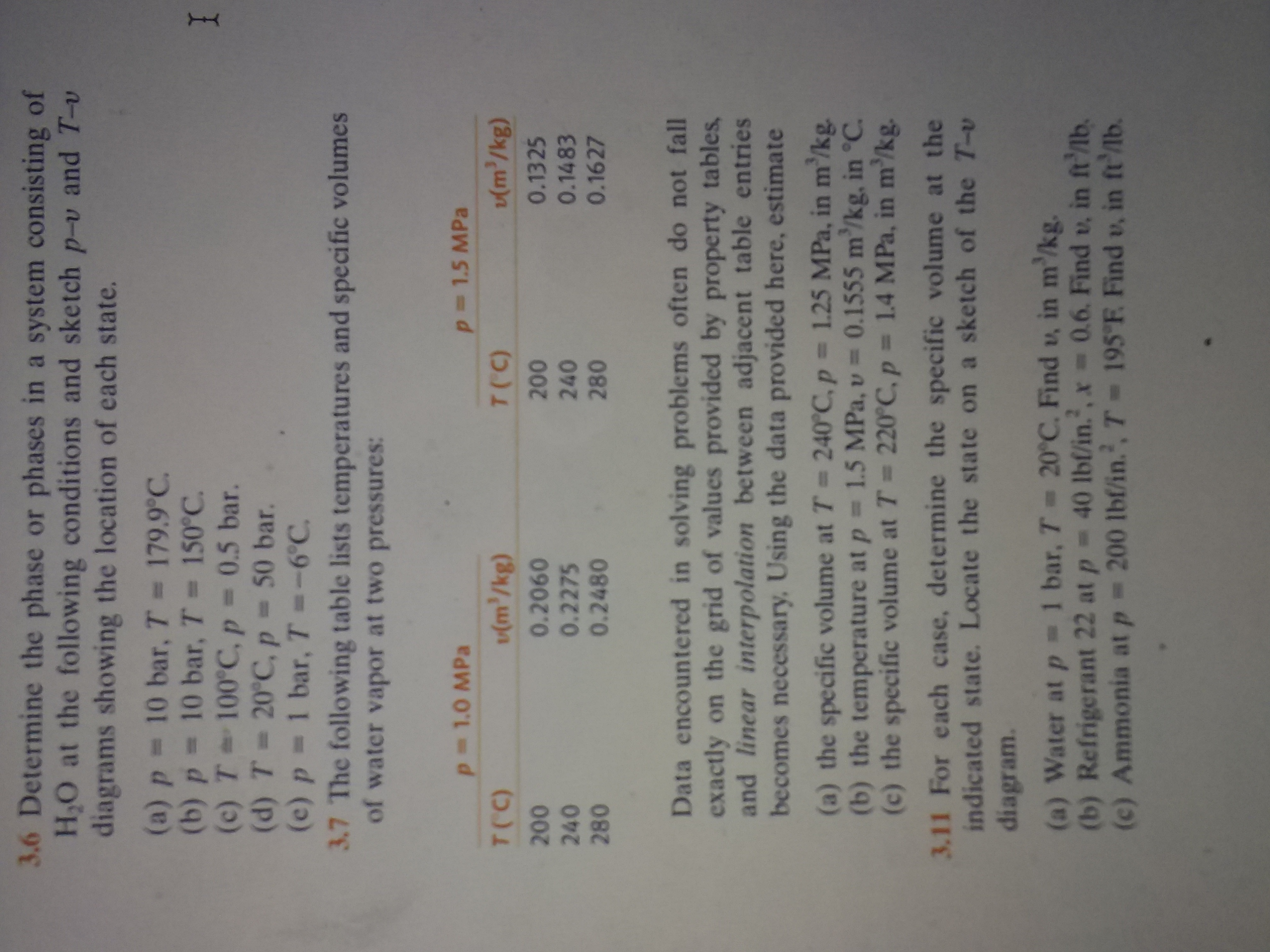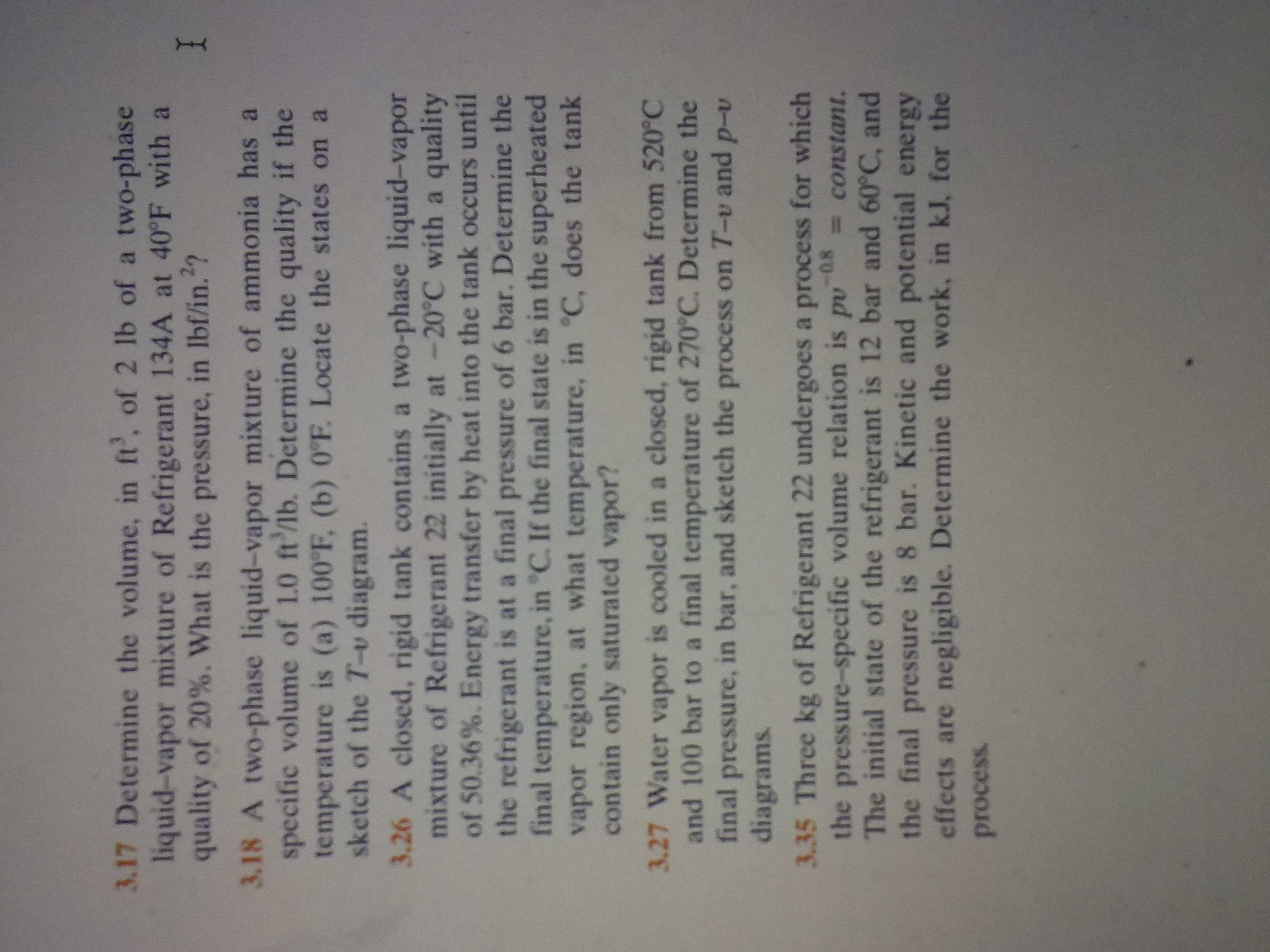# 3.6 Determine the phase or phases in a system consisting of H.O at the following conditions and sketch p-v and T-v diagrams showing the location of each state. (a) p 10 bar, T = 179.9°C (b) p 10 bar, T= 150°C (c) T 100 C, p = (d) T 20°C, p (e) p 1 bar, T =-6°C. 3.7 The following table lists temperatures and specific volumes of water vapor at two pressures: I = 0.5 bar. - 50 bar. p 1.5 MPa p 1.0 MPa (m/kg) (m'/kg) T(C) T(C) 0.1325 200 0.2060 0.2275 0.2480 200 0.1483 240 280 240 280 0.1627 Data encountered in solving problems often do not fall exactly on the grid of values provided by property tables and linear interpolation between adjacent table entries becomes necessary, Using the data provided here, estimate (a) the specific volume at T 240°C, p 1.25 MPa, in m'/kg. (b) the temperature at p 1.5 MPa, v 0.1555 m/kg, in °C. (c) the specific volume at T 220°C, p 1.4 MPa, in m'/kg. 3.11 For each case, determine the specific volume at the indicated state. Locate the state on a sketch of the T-v diagram. (a) Water at p 1 bar, T 20°C. Find u, in m'/kg. (b) Refrigerant 22 at p 40 lbl/in.', x = 0.6. Find v, in ft'lb. (c) Ammonia at p = 200 lbf/in., T= 195"F Find v, in ft'lb. 3.17 Determine the volume, in ft', of 2 lb of a two-phase liquid-vapor mixture of Refrigerant 134A at 40°F with a quality of 20%. What is the pressure, in Ibf/in.? I 3.18 A two-phase liquid-vapor mixture of ammonia has a specific volume of 1.0 ft'/lb. Determine the quality if the temperature is (a) 100°F, (b) 0°F. Locate the states on a sketch of the T-v diagram. 3.26 A closed, rigid tank contains a two-phase liquid-vapor mixture of Refrigerant 22 initially at -20°C with a quality of 50.36%. Energy transfer by heat into the tank occurs until the refrigerant is at a final pressure of 6 bar. Determine the final temperature, in °C. If the final state is in the superheated vapor region, at what temperature, in °C, does the tank contain only saturated vapor? 3.27 Water vapor is cooled in a closed, rigid tank from 520°C and 100 bar to a final temperature of 270C. Determine the final pressure, in bar, and sketch the process on T-v and p-v diagrams 3.35 Three kg of Refrigerant 22 undergoes a process for which the pressure-specific volume relation is pv The initial state of the refrigerant is 12 bar and 60°C, and the final pressure is 8 bar. Kinetic and potential energy effects are negligible. Determine the work, in kJ, for the -0.8 = constant. process

Questionhelp_outlineImage Transcriptionclose3.6 Determine the phase or phases in a system consisting of H.O at the following conditions and sketch p-v and T-v diagrams showing the location of each state. (a) p 10 bar, T = 179.9°C (b) p 10 bar, T= 150°C (c) T 100 C, p = (d) T 20°C, p (e) p 1 bar, T =-6°C. 3.7 The following table lists temperatures and specific volumes of water vapor at two pressures: I = 0.5 bar. - 50 bar. p 1.5 MPa p 1.0 MPa (m/kg) (m'/kg) T(C) T(C) 0.1325 200 0.2060 0.2275 0.2480 200 0.1483 240 280 240 280 0.1627 Data encountered in solving problems often do not fall exactly on the grid of values provided by property tables and linear interpolation between adjacent table entries becomes necessary, Using the data provided here, estimate (a) the specific volume at T 240°C, p 1.25 MPa, in m'/kg. (b) the temperature at p 1.5 MPa, v 0.1555 m/kg, in °C. (c) the specific volume at T 220°C, p 1.4 MPa, in m'/kg. 3.11 For each case, determine the specific volume at the indicated state. Locate the state on a sketch of the T-v diagram. (a) Water at p 1 bar, T 20°C. Find u, in m'/kg. (b) Refrigerant 22 at p 40 lbl/in.', x = 0.6. Find v, in ft'lb. (c) Ammonia at p = 200 lbf/in., T= 195"F Find v, in ft'lb. fullscreenhelp_outlineImage Transcriptionclose3.17 Determine the volume, in ft', of 2 lb of a two-phase liquid-vapor mixture of Refrigerant 134A at 40°F with a quality of 20%. What is the pressure, in Ibf/in.? I 3.18 A two-phase liquid-vapor mixture of ammonia has a specific volume of 1.0 ft'/lb. Determine the quality if the temperature is (a) 100°F, (b) 0°F. Locate the states on a sketch of the T-v diagram. 3.26 A closed, rigid tank contains a two-phase liquid-vapor mixture of Refrigerant 22 initially at -20°C with a quality of 50.36%. Energy transfer by heat into the tank occurs until the refrigerant is at a final pressure of 6 bar. Determine the final temperature, in °C. If the final state is in the superheated vapor region, at what temperature, in °C, does the tank contain only saturated vapor? 3.27 Water vapor is cooled in a closed, rigid tank from 520°C and 100 bar to a final temperature of 270C. Determine the final pressure, in bar, and sketch the process on T-v and p-v diagrams 3.35 Three kg of Refrigerant 22 undergoes a process for which the pressure-specific volume relation is pv The initial state of the refrigerant is 12 bar and 60°C, and the final pressure is 8 bar. Kinetic and potential energy effects are negligible. Determine the work, in kJ, for the -0.8 = constant. process fullscreen